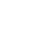### MAT-102INTRODUCTION TO PROBABILITY AND STATISTICS 3 Credits

This course is a part of:
Associate's Degree Program
Bachelor's Degree Program

This course will give the student a working knowledge of the most important basic concepts of probability and statistics. The course will teach the methods by which data is sorted, characterized, visualized and interpreted. The course will discuss important probability concepts such as events, sample spaces, conditional probability and the effects of multiple variables. The course will also focus on statistical distributions, sample sizes and testing. Finally, the course will touch on regression analysis and other more complex statistical analysis.

At the completion of this course, the student will have acquired the ability to:

• Apply the Fundamental Counting Principle.
• Explain the mean, median, and mode of a population and of a sample.
• Discuss if a distribution is a probability distribution.
• Apply the Central Limit Theorem to find the probability of a sample mean.
• Analyze the minimum sample size required when estimating a population proportion.
• Identify type I and type II errors and interpret the level of significance.
• Discuss whether two samples are independent or dependent.
• Organize a t-test to test the mean of the differences for a population of paired data.
• Perform a hypothesis test for a population correlation coefficient ρ.
• Select a chi-square distribution to test whether two variables are independent.
• Analyze the Wilcoxon rank sum test to determine if two independent samples are selected from populations having the same distribution.